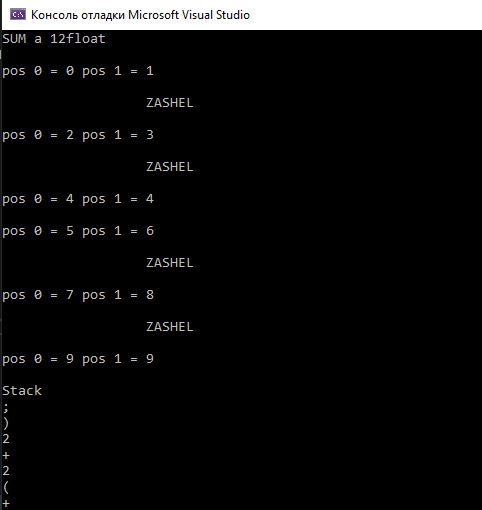Why ReSharper says that code is unreachable, but its working fine?`int pos = { 0,0 };for (auto element : str){for (int i = 0; i < operandsSize; i++){if (element == operands[i]){std::string tempstr;pos = str.find(element,pos);std::cout << std::endl << "pos 0 = " << pos << " pos 1 = " << pos << std::endl;int var = pos;if (pos != pos) {//unreachabledo{tempstr += str[var];std::cout << std::endl << " ZASHEL " << std::endl;var++;} while (var < pos);//unreachable}if (!tempstr.empty())varsAndOps.push(tempstr);tempstr = element;if (!tempstr.empty())varsAndOps.push(tempstr);pos = pos + 1;}}}`

1 commentHello,

This is probably the same issue as https://youtrack.jetbrains.com/issue/RSCPP-28763, should get fixed in 2020.1 EAP 3.